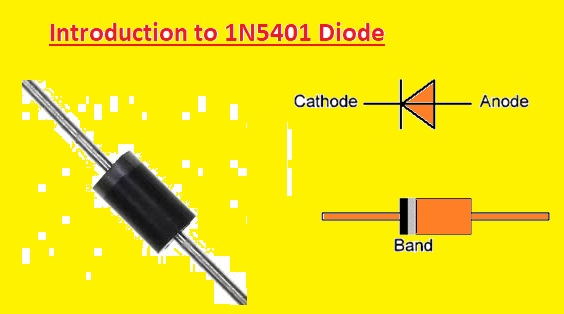Hello, readers welcome to the new post. In this post, we will discuss the Introduction to 1N5401 Diode. The diode is a two-terminal electronic component used in circuits as rectifiers and switches. It has anode and cathode pins that are positive and negative according to the configuration. The diode is used to convert AC current into Dc current since its works in forward biased state and blocks current in a reverse biased.

There are different types of diodes used in electronics. In this post, we will discuss the structure, applications, and other parameters of the 1N5401 Diode. So let’s get started Introduction to 1N5401 Diode

### Introduction to 1N5401 Diode

• 1N5401 is a standard recovery rectifier diode that is used in power supply circuits.  This diode comes in dual inline 201 packagings.
• Its main feature is that it has the ability to run load up to three campers.
• Its advantages are that it offers less reverse current leakage, highly reliable operation, high efficiency, and the ability to surge current.
• 1N5401 is part of 1N540X didoe series. This series consists of nine diode that are  1N5404, 1N5405, 1N5406,1N5400, 1N5402, 1N5403, 1N5407 and 1N5408
• These diodes have different repetitive peak reverse voltage and the same ratings
• The value of repetitive peak reverse voltage value is fifty to 1000 volts. The low volts can be fined through diode title or number
• For instance, 1N5400 has the lowest repetitive reverse voltage of fifty volts, and for 1N5401 100 volts, etc

### 1N5401 Features

• Some features of 1N5401 are listed here
• Its main feature is that has a high curent and a small structure
• It can handle high-surge current
• Its forward voltage drop is less
• Its casing is plastic packaging
• It comes in volume structures
• The highest peak reverse voltage value is 100 volts
• The highest average forward output curent is three ampers
• The highest forward voltage loss is 1.0A DC to 1.1  volts
• The lowest forward output current is three ampers
• Junction capacitance is forty picofarad
• Diode packaging is DO-27
• The weight of the diode is 1.18G
• The operating temperature is -65 to+175 °### 1N5401 Specifications

• Its main specifications are discussed here
• It comes in tin plating
• It has through hole mounting type
• It has two pinouts
• Its weight is about 4.535924g
• Moisture Sensitivity Level (MSL) is 1
• It has Min Operating Temperature value  -65°C
• Voltage – Rated value is DC 100V
• The base Part Number is 1N5401
• Component Configuration is Single
• It is a standard type of diode
• The power dissipation value is  6.25W
• Voltage – Forward (Vf) (Max) is If 1.2V and current is  3A
• Operating Temperature is Junction -55°C~150°C
• Voltage DC Reverse (Vr) (Max) is 100V
• The forward Voltage value is 1.2V
• Average Rectified Current is 3A
• Max Repetitive Reverse Voltage (Vrrm) value is 100V
• Peak Non-Repetitive Surge Current is 200A
• Maximum Forward Surge Current (Ifsm) is 200A
• Its dimension are Height is  5.6mm and width are 5.6mm
• It comes in Package / Case DO-201AD, Axial
• Part Status is Obsolete
• Max Operating Temperature is 125°C
• Capacitance is 30pF
• The current Rating is 3A

### Where to use 1N5401

• This diode is configured for rectifier circuits so it is used in inverter circuits, and power supply converters. It is also part of blocking voltage circuits, provides protection to components switching circuits and is used for some general-purpose operations

### 1N5401 Applications

• It is used for protecting circuits
• Chargers
• Power supply
• Ac to dc converters
• Low to high volts rectification
• Step-up and step-down converters
• Used for blockages of volts

#### Related Posts

That is all about the 1N5401 Diode all details has explained. If you have any questions ask them here